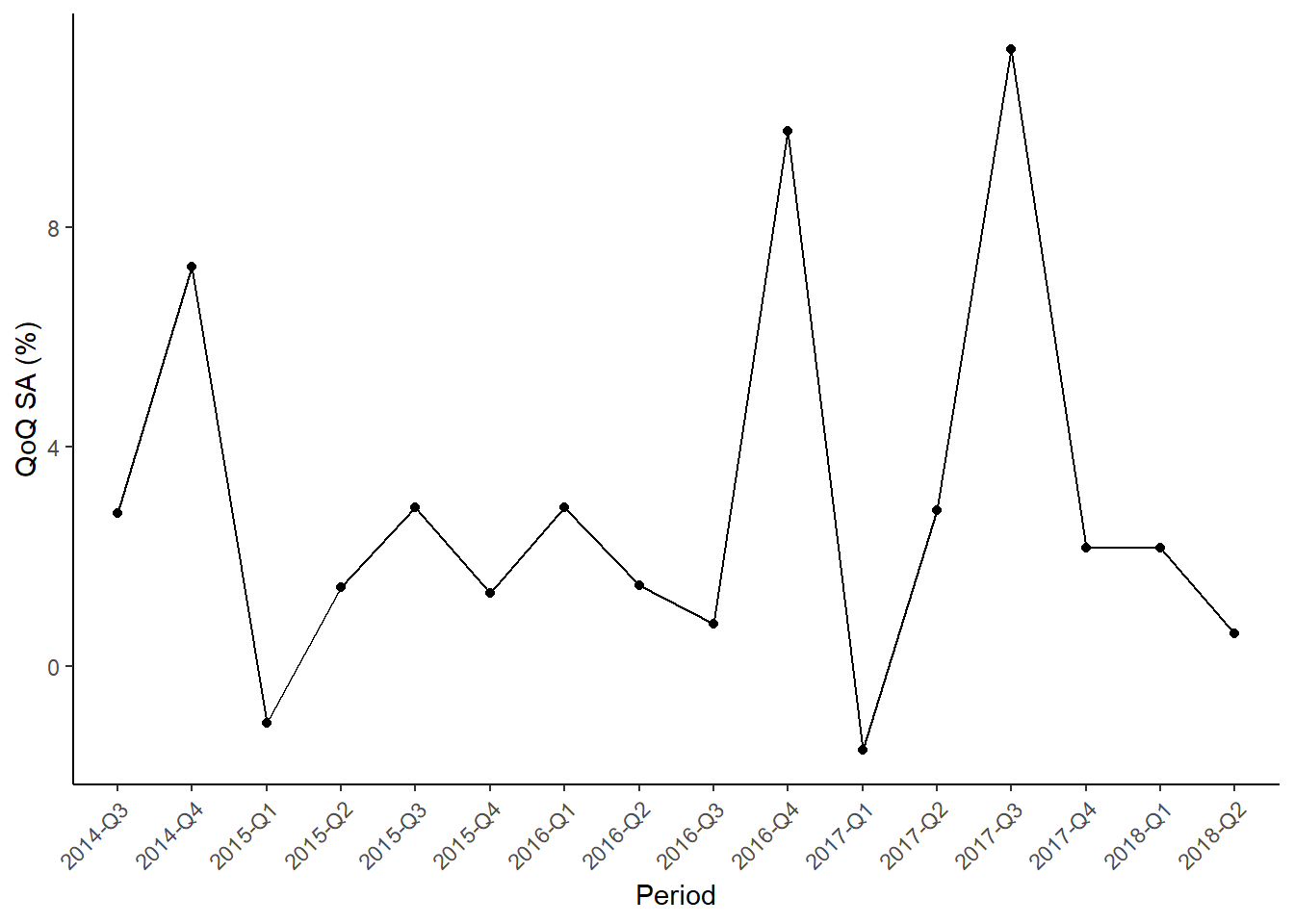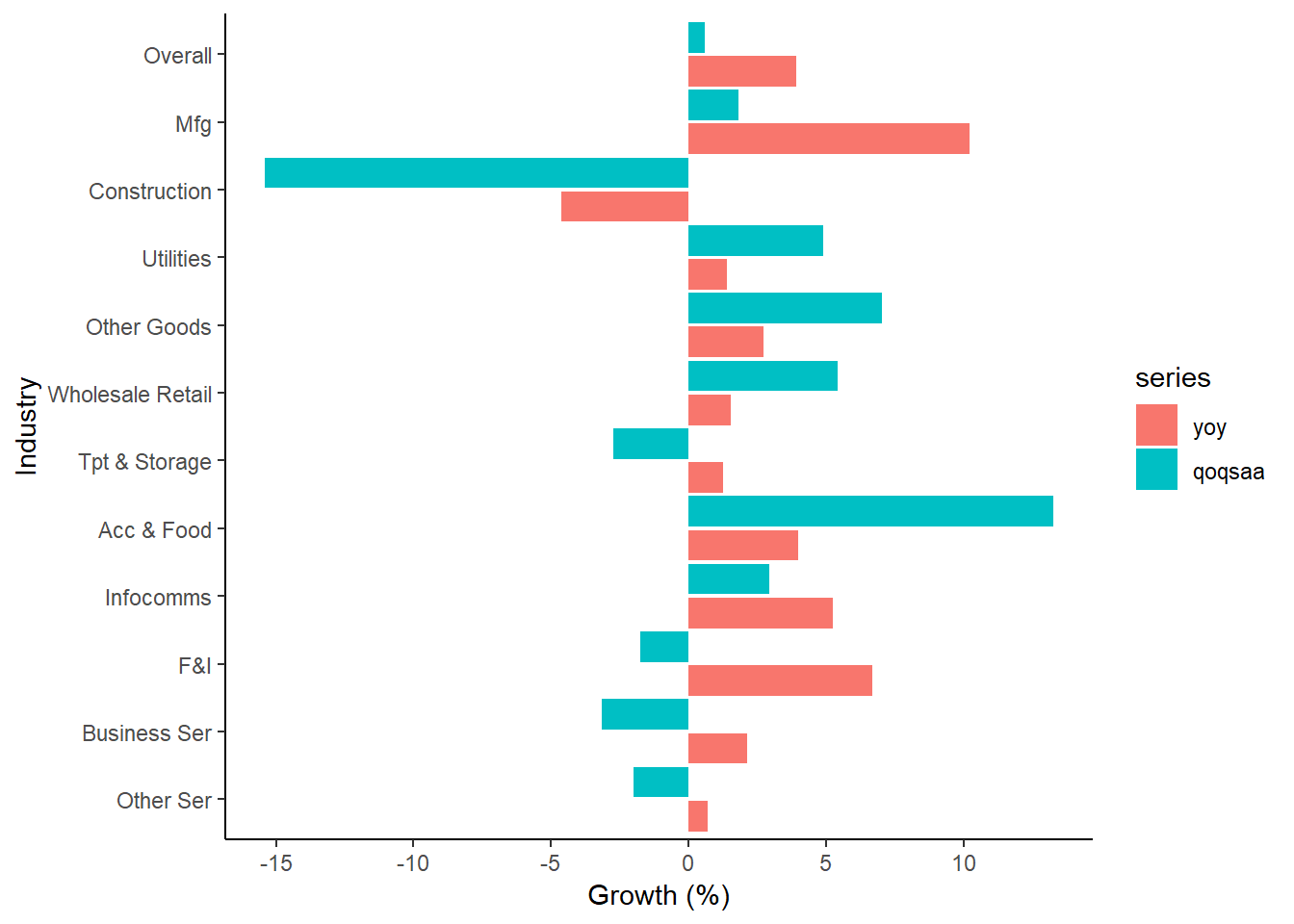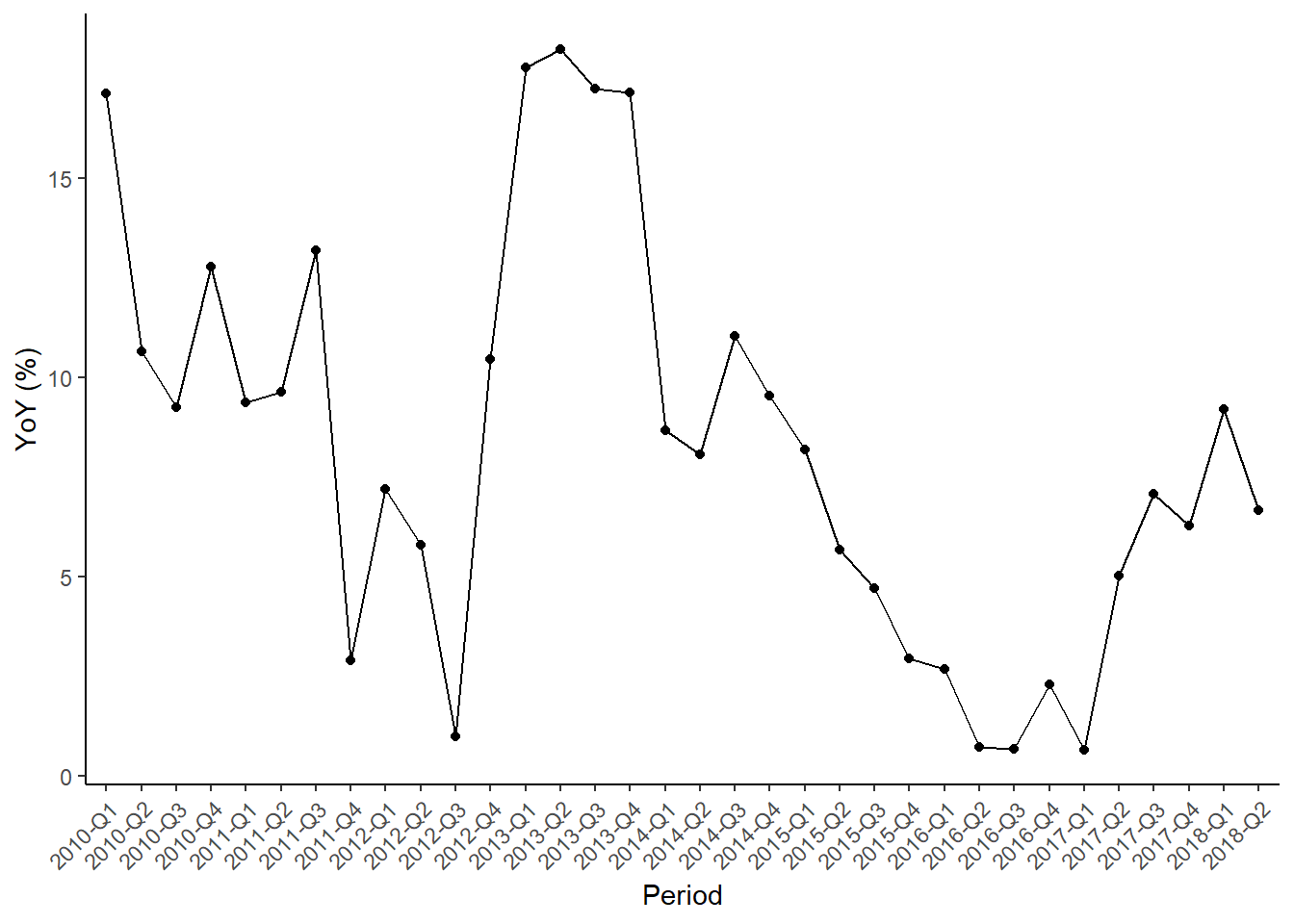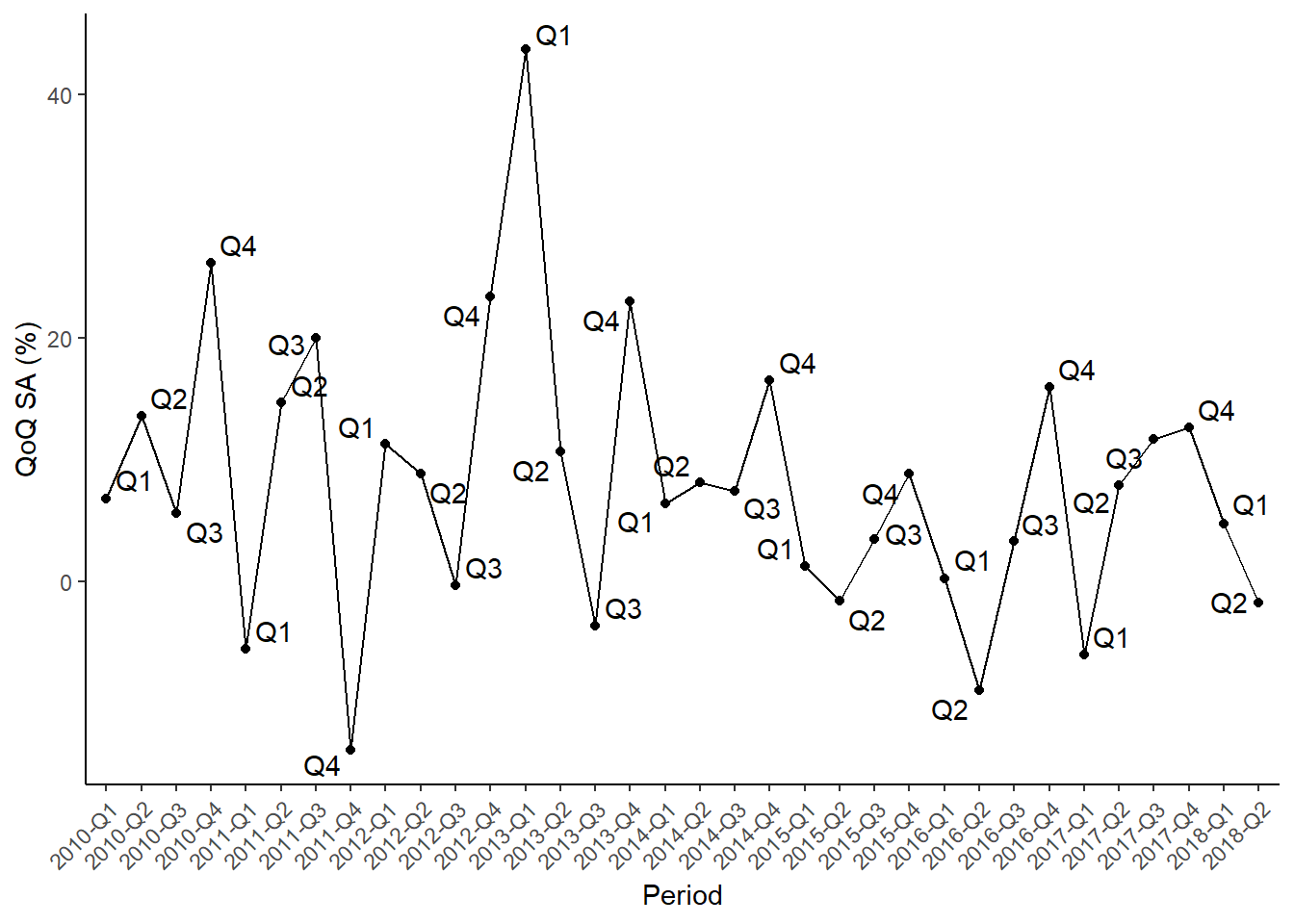I have been trying to catch-up on the latest release of Singapore’s economic results. Unfortunately, the official press release or media reports are not very useful. They either contain too much irrelevant information or not enough details for my liking. Maybe I just like looking at the numbers and letting the figures speak for themselves. Hence, I decided to obtain the data from the official SingStat’s Table Builder website. Turns out that they have an API available that provides data for selected data series including the GDP figures.

This makes it easy to obtain the required data without having to navigate through the complicated tree structure of table builder. The best part about having an API is that I can just re-run the same set of codes and produce my own customise GDP report tailored to my liking! For the first technical post of this blog, I decided to do a write-up on using the API to extract GDP figures and generate some graphs in R. A more detailed set of codes is available on github.

I use the httr package to read in JSON data before manipulating the data in dplyr and reshape2. ggplot2 is used for graphing and to add a little interactivity we can also play around with the plotly package. First, we have to read in the JSON data and convert it to string format. I decided to read in both the seasonally-adjusted VA figures and raw VA figures as they are used to calculate quarter-on-quarter and year-on-year growth respectively.

library(httr)
library(reshape2)
library(dplyr)
library(ggplot2)

url_va_sa  <- "http://www.tablebuilder.singstat.gov.sg/publicfacing/api/json/title/12411.json"
url_va  <- "http://www.tablebuilder.singstat.gov.sg/publicfacing/api/json/title/12407.json"

raw.result <- GET(url=url_va_sa)
names(raw.result)
##   "url"         "status_code" "headers"     "all_headers" "cookies"
##   "content"     "date"        "times"       "request"     "handle"
raw.content <- rawToChar(raw.result$content) # Convert raw bytes to string raw.content <- content(raw.result) # levels correspond to the statistics level of aggregation # 1 - overall economy, 2 - goods / services, 3 - sector level Browsing the raw.content variable, we can see that it is a messy list of lists. names(raw.content) ##  "Level1" "Level2" "Level3" ##  "Note" "VariableFootNotes" "Unit" ##  "Datesource" "GeneratedBy" "DateGenerated" ##  "Contact" Level1, Level2, Level3 contain the data we are interested in at various levels of industry aggregation. Let’s convert it to a more managable dataframe format and extract the required data. The do.call function along with lapply helps to unpack the list. data <- do.call(what = "rbind", args = lapply(raw.content$Level1, as.data.frame))
data.sector <- do.call(what = "rbind", args = lapply(raw.content$Level3, as.data.frame)) data$value <- as.numeric(as.character(data$value)) data.sector$value <- as.numeric(as.character(data.sector\$value))
data <- data[,c("quarter","value")]
data.sector <- data.sector[,c("quarter","value","level_3")]
names(data)[names(data) == 'quarter'] <- 'period'
names(data.sector)[names(data.sector) == 'quarter'] <- 'period'
names(data.sector)[names(data.sector) == 'level_3'] <- 'industry'

Now we can use the levels data and calculate the quarter-on-quarter seasonally-adjusted annualised growth rate (qoqsaa).

data <- data %>% mutate(qoqsaa = ((value/lag(value))^4-1)*100)
data.sector <- data.sector %>% group_by(industry) %>%
mutate(qoqsaa = ((value/lag(value))^4-1)*100) %>% ungroup()

We can repeat the process for the non-seasonally-adjusted data and calculate the year-on-year growth rates.[Code omitted due to repetition.]

Let’s take a look at some plots of the data.

ggplot(data[(nrow(data)-16+1):nrow(data),], aes(period, qoqsaa, group=1)) +
geom_point() + geom_line() + ylab("QoQ SA (%)") + xlab("Period") + theme_classic() +
theme(axis.text.x=element_text(angle=45,hjust=1,vjust=1))Figure 1: QoQ GDP Trend

And here’s a plot comparing qoq and yoy growth for all the industries:Wondering what the actual percentage change is? We can convert the graph into a more interactive one using a simple line of code. And since it is locally generated, it can be hosted on github as well.

While the precision of our calculated data is satisfying, the scale of the graph is being skewed by one particular sector. The other goods sector (Agriculture and Fishing, Mining and Quarrying) contracted 16.38% yoy (yikes!).1 The other goods sector is normally not given any coverage as it is a very small component of GDP (just 30m). Could the decline be mostly due to noisy fluctuations?

other.goods.data <- va.sector.comb %>% filter(year>=2012 & industry=="Other Goods Industries")                     %>% select(period, yoy, qoqsaa) %>% melt(measure.vars=c("yoy","qoqsaa"))

other.goods.plot<-ggplot(other.goods.data, aes(period, value, group=variable, color=variable)) + geom_point() + geom_line() + ylab("QoQ SA (%)") + xlab("Period") + theme_classic() +        theme(axis.text.x=element_text(angle=45,hjust=1,vjust=1))

Not suprisingly there is a downward trend in the sector, but the recent quarter’s dip is the sharpest in all 4 years that I have plotted. The drastic decline in quarter-on-quarter VA figures for the finance and insurance industry is of potentially greater concern. Let’s take a closer look by plotting an extended time series.Figure 2: yoy plotFigure 2: qoq plot

Interestingly, from the yoy data it appears that the contribution of finance and insurance to Singapore’s GDP growth is plateauing off. The qoq data is even more suprising showing a strong cyclical pattern since 2014. This is of particular concern as the F&I figures are not seasonally-adjusted. DOS believes that the sector does not exhibit seasonality, but looking at the recent data, a case could be made that the fundamentals of the sector has changed substantially over the past 3 years and one should definitely treat the qoq figures extremely cautiously.

1. I manually verified the figures with the official ones due to this huge number.